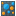#Mutual Information Matrix (RapidMiner Studio Core)

## Synopsis

This operator calculates the mutual information between all attributes of the input ExampleSet and returns a mutual information matrix. Mutual information of two attributes is a quantity that measures the mutual dependence of the two attributes.

## Description

Mutual information is one of many quantities that measures how much one attribute tells us about another. It is a dimensionless quantity, and can be thought of as the reduction in uncertainty about one attribute given the knowledge of another. High mutual information indicates a large reduction in uncertainty; low mutual information indicates a small reduction; and zero mutual information between two attribute means the variables are independent.

This operator calculates the mutual information matrix between all attributes of the input ExampleSet. Please note that this simple implementation performs a data scan for each attribute combination and might therefore take some time for non-memory tables.

## Input

•example set (Data Table)

This input port expects an ExampleSet. It is the output of the Retrieve operator in the attached Example Process. The output of other operators can also be used as input.

## Output

•example set (Data Table)

The ExampleSet that was given as input is passed after some modifications to the output through this port. Please note that this ExampleSet is not exactly the same as the input ExampleSet.

•matrix (Numerical Matrix)

The mutual information of all attributes of the input ExampleSet are calculated and the resultant matrix is returned from this port.

## Parameters

• number_of_binsThis parameter specifies the number of bins to be used for numerical attributes. Range: integer

## Tutorial Processes

### Mutual information matrix of the Polynomial data set

The 'Polynomial' data set is loaded using the Retrieve operator. A breakpoint is inserted here so that you can view the ExampleSet. You can see that the ExampleSet has 5 real attributes. The Mutual Information Matrix operator is applied on this ExampleSet. The resultant matrix can be viewed in the Results Workspace.